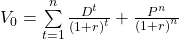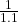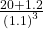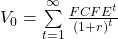Equity Valuation: Concepts and Basic Tools | IFT World
IFT Notes for Level I CFA® Program
IFT Notes for Level I CFA® Program

# Part 1

## 1.  Introduction

We began the equities section with a discussion on how securities markets are organized, how efficient markets are, the different types of equity securities, and how to analyze an industry and a company. The focus of this reading is on determining the intrinsic value of the security.

## 2.  Estimated Value and Market Price

The intrinsic value of a security is based on its fundamentals and characteristics. It is also called the fundamental value or estimated value as it is based on the fundamentals such as earnings, sales, and dividends. If the intrinsic value is different from the market price, then you are implicitly questioning the market’s estimate of value.

Assume, Caterpillar Inc. is trading on NYSE at $84.53. An analyst estimates its intrinsic value as$88.21. Is it overvalued, fairly valued, or undervalued? Going by the relationships given above, the security is undervalued. In reality, making this decision is not that straightforward. It depends on an analyst’s input values and assumptions in the model. Some factors to consider when market value  intrinsic value:

• Percentage difference between the market price and intrinsic value. Assume you calculate the intrinsic value of a security to be $95, but it is currently trading at$180. Since the percentage difference is large, it is prudent to calculate the intrinsic price once again because the assumptions or input data to the model may be incorrect.
• Confidence in your model. High confidence means the market price will converge to the intrinsic value over the time horizon considered. If your confidence is low, you might see the two prices diverging substantially.
• Model sensitivity to assumptions. If many securities appear to be under- or overvalued, analysts should check the model’s sensitivity to their inputs.
• Number of analysts. The more the number of analysts covering a security, the less the mispricing. Recollect what we read about efficient markets. The market price, in this case, is likely to reflect intrinsic value. Securities neglected by analysts are often mispriced.

## 3. Categories of Equity Valuation Models

Three major categories of equity valuation model are:

Present value models

• They estimate value as present value of expected future benefits.
• Future benefits are defined as either cash distributed to shareholders (dividend discount models) or cash available to shareholders after meeting the necessary capital expenditure and working capital expenses (free-cash-flow-to-equity models).

Multiplier models

• They estimate intrinsic value based on a multiple of some fundamental variable.
• For example, either Stock price / earnings (or sales, book value, cash flow).
• Or Enterprise value / EBITDA (or sales).

Asset-based valuation models

• They estimate the value of equity as the value of assets less the value of liabilities.
• Book values of assets and liabilities are typically adjusted to their fair values when using these models.

The choice of model depends on availability of information and the analyst’s confidence in the appropriateness of the model. Generally, analysts will try to use more than one model.

## 4. The Background for the Dividend Discount Model

### 4.1 Dividends: Background for the Dividend Discount Model

A dividend is a distribution made to shareholders based on the number of shares owned.

Cash dividends are payments made to shareholders in cash. The three types of cash dividends are:

1. Regular cash dividends: They are paid out on a consistent basis. A stable or increasing dividend is viewed as a sign of financial stability.
2. Special dividends: They are one-time cash payments when the situation is favorable (Also called as extra dividends or irregular dividends; used by cyclical firms).
3. Liquidating dividend: This is distributed to shareholders when a company goes out of business.

Stock dividend: Company distributes additional shares instead of cash. A stock dividend simply divides the ‘pie’ (the market value of equity) into smaller pieces without affecting the value of the pie. Since the market value of equity is unaffected, stock dividends are not relevant for valuation purposes.

Stock split: Increases the number of shares outstanding. For example, in a 2 for 1 split, each shareholder is issued an additional share for each share currently owned.

Reverse stock split: Reduces the number of shares outstanding. For example, in a 1 for two reverse stock split, each shareholder would receive one share for every two old shares.

Stock splits and reverse stock split are similar to stock dividends. They do not change the market value of equity hence they are not relevant for valuation purposes.

Share repurchase: This is an alternative to cash dividends. Here the company uses cash to buy back its own shares. An important point to note is that, as compared to stock dividends and stock splits, share repurchases affect the market value of equity. The effect on shareholders’ wealth is equivalent to a cash dividend. Some key reasons why companies engage in share repurchases instead of cash dividends are:

1. to support share prices.
2. flexibility in the amount and timing of cash distribution.
3. when tax rates on capital gains are lower than tax rates on dividends.
4. to offset the impact of employee stock options.

Dividend payment chronology

A dividend payment schedule is as follows:

1. Declaration date: Company declares the dividend.
2. Ex-dividend date: Cutoff date on or after which buyers of a stock are not eligible for the dividend. Also is the first date when the stock trades without dividend.
3. Holder-of-record date: A record of shareholders who are eligible to receive the dividend is made (usually two days after the ex-dividend date).
4. Payment date: Dividend payment made to the shareholders.

## 5. Dividend Discount Model (DDM) and Free-Cash-Flow-to-Equity Model (FCFE)

This model is based on the principle that the value of an asset should be equal to the present value of the expected future benefits. The simplest present value model is the dividend discount model (DDM). According to DDM, the intrinsic value of a stock is the present value of future dividends plus the present value of terminal value.

Intrinsic value = PV of future dividends + PV of terminal valueExample

For the next three years, the annual dividends of stock X are expected to be 1.0, 1.1, and 1.2. The expected stock price at the end of year 3 is expected to be \$20.00. The required rate of return on the shares is 10%. What is the estimated value?

Solution:

Calculate the present value of each of the future dividends at the reqd. rate of return of 10%.

PV of cash flow 1 == 0.909
PV of cash flow 2 == 0.909
PV of cash flow 3 == 15.928

Estimated value = 0.909 + 0.909 + 15.92 = 17.74

In the exam, use a financial calculator with the following keystrokes:

CF0 = 0; CF1 = 1; CF2 = 1.1; CF3 = 21.2; I = 10%, CPT NPV

NPV = 17.7

Free cash flow to equity (FCFE) is the residual cash flow available to be distributed as dividends to common shareholders. In practice, FCFE model is often used because:

• FCFE is a measure of a firm’s dividend-paying capacity.
• It can be used for a non-dividend paying stock (unlike DDM which requires the timing and the amount of the first dividend to be paid).
• It can also be used for a company that pays dividends which are extremely small or the dividends being paid are not an indication of a company’s ability to pay dividends.
• Not all of the available cash flow is distributed to shareholders because a company retains some part of it for future investments as a going concern.

FCFE = CFO – FCInv + Net borrowing
where: FCInv = fixed capital investment

Net borrowing = borrowings – repaymentsRequired Rate of Return on a share

Analysts generally use CAPM (capital asset pricing model) to calculate the required return on a share.

Required rate of return on share = current expected risk free rate + Betai [market risk premium]

In addition to CAPM, there are other methods to calculate the required return like the bond yield plus risk premium method which we will see later.